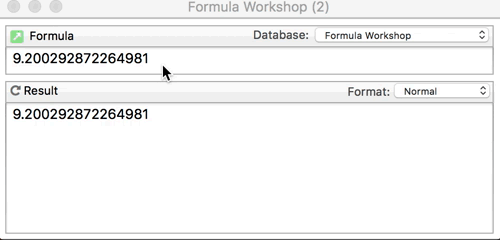# Difficulty with Round()

Round(MyNumber, 0.01) should remove any digits after the hundredth’s of a cent, right? So help me understand what’s happening here. Where do the extra digits come from?

![image|601x500]

The destination field should be Number (float) and the Output Pattern should be #.## (or \$#,.## if you have large numbers and wish to retain the currency symbol).

… and if you use the output pattern, you don’t need the round( formula.

The reason for those results is: The round( function does not work like a text funnel (that removes some digits after the hundredth), it is binary Math that calculates that value (with appropriate rounding up or down).

As the question is not about Output Patterns, nor currency symbols, I’ll leave that part aside.

While .3333 does round, 6.10 does not due to the .1 not representing easily in Binary because .1 is a prime.
See Decimal and Binary Numbers for a longer answer.

Floating point numbers are precise to 53 significant binary digits. This is approximately equivalent to 16 significant decimal figures, but not quite.

For any given order of magnitude, there will be a few more 16 digit decimal numbers than there are 53 digit binary numbers, so there will be cases where two consecutive 16 digit decimals convert to the same 53 digit binary. When you convert back to decimal, the one that comes closest to the binary value won’t necessarily be the same decimal you started with.The origin and destination fields are both floating point. I don’t care about the output pattern right now because I’m comparing numbers, not text.

My programming does a final double-check of my Inventory calculations prior to running our Profit & Loss statements. So if `round(MyNumber, .01)` does not really actually round off numbers to 2 digits past the decimal point, it sounds like you’re saying that there could be instances where, say, `round(3, .01) + round(4, .01)` does not equal `round(7, .01)`. If that’s true, then it seems like I can’t count on my double-check to work right. Correct?

Yes. That’s true, but in nearly every case, you should expect

``````pattern(3+4,"#.##") to equal pattern(7,"#.##")
``````

You can also check to see if the difference is less than something like 0.001 . If you have two values that differ by less than one tenth of a cent, then they are, for all intents and purposes, equal.

Many fractional values cannot be represented in a finite floating point value. For example, consider the fraction 1/3. In a decimal notation, this is:

``````0.3333333333333333...
``````

The … at the end indicates that this is an infinite sequence. In a base 10 numeric system, there is no way to express the value 1/3 in a finite number of digits.

Almost all modern software use a base 2 numeric system, aka binary. Just as in base 10, there are many fractional values that cannot be exactly expressed in a finite number of digits. Since we humans are used to dealing with base 10, this can produce what seem to be unusual results, for example values that can easily be expressed in decimal like 1/10 and 1/5 cannot be exactly expressed as binary values in a finite number of digits. The IEEE floating point system used by Apple allows for up to 52 binary digits (bits) of precision, which is a lot, but a long way from infinite. (It’s not just Apple, this is an industry standard).

So the problem is that when you round 9.97 to the nearest 0.01, you are producing a value that is not exactly representable as a IEEE floating point. The system comes up with the nearest possible binary value, but when displayed as a decimal value you’ll see that it isn’t the exact decimal value you might have expected.

For comparing two fractional values for equality, the techniques Dave suggested are good – either using the pattern( function and comparing the text values:

``````pattern(X,"#.##") = pattern(Y,"#.##")
``````

or checking if the difference is less than a small value:

``````abs(X-Y)<0.01
``````

One thing I noticed in the original post is that the Morph dialog doesn’t use the field output pattern for the previewed result of the morph. This doesn’t really have anything to do with your question, but it probably should use the pattern. So I’m filing that for later attention.